## Simple Diagnostic Biosphere Model (SDBM)The model is based on the approach of Monteith[ 1972, 1977] and Kumar and Monteith [ 1981].It considers in stantaneous NPP (called P in the formulae) as a fraction of the incoming solar energy stored in to organic dry matter.

carbon cycle data assimilationterrestrial biosphereuncertainty analysis
23

#### Contributor#### Authorship

Authorship is unclear, you can claim the item.

#### Classification(s)

Earth System SubjectEarth Surface System Synthesis

#### Detailed Description

In brief,the model calculates a pure seasonal cycle of net biosphere–atmosphere fluxes as the difference of two fluxes inany month at each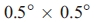land grid cell,

F = H - NPP

where F is the net flux to the atmosphere, H is the heterotrophic respiration, and NPP is the net primary productivity.

The calculation is performed on the basis of measured Normalized Differential Vegetation Index (NDVI), incoming solar radiation, and surface temperature. There is an additional time-dependent water-stress term a, which may limit the efficiency either of NPP, or heterotrophic respiration, or both. This factor will be included in various of the calculations in this work in either of the two fluxes H and NPP; in the case described below, it is included in both.

NPP is calculated for each month as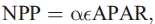where APAR is absorbed photosynthetically active radiation and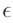is a light-use efficiency parameter. APAR is computed from NDVI data by Gallow  and incoming solar radiation inferred from cloudiness data by Leemans and Cramer  using the method of Linacre .

Heterotrophic respiration H is calculated as an exponential function of temperature as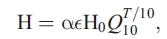where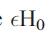is the heterotrophic respiration rate at T = 0 and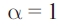(i.e., no water stress) and Q10 is the ratio of respiration at T + 10 to that at T; T is given in °C. We have absorbedinto the formulation of H for later convenience.

In order to ensure a balanced biosphere (no net annual flux), we rescale H0 at each grid cell so that integrated NPP and H are equal over a year. Thus H0 is not a free parameter.

The water-stress factor, a = AET/PET (actual divided by potential evapotranspiration), is computed with the model of Prentice et al. . PET is assumed equal to equilibrium evapotranspiration, and AET is the minimum of PET and a supply rate assumed proportional to soil moisture. The model neglects the effect of soil freezing and snow accumulation, so that at low ambient temperatures where PET is mostly small, a tends toward 1. Freezing-induced drought effects are therefore not considered in SDBM; low temperatures rather affect H through its temperature dependence given by the value of Q10.

We can now write the total flux as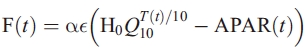Subject to the constraint that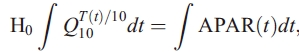where integrals are taken over the annual cycle. Integrals are calculated as sums of monthly values weighted by the length of the month.

#### How to cite

mimi (2019). Simple Diagnostic Biosphere Model (SDBM), Model Item, OpenGMS, https://geomodeling.njnu.edu.cn/modelItem/7882aef5-568d-4b1a-811b-86cae1cf0557

#### Comment(s)

You can link related `{{typeName}}` from your personal space to this model item, or you can create a new {{typeName.toLowerCase()}}.